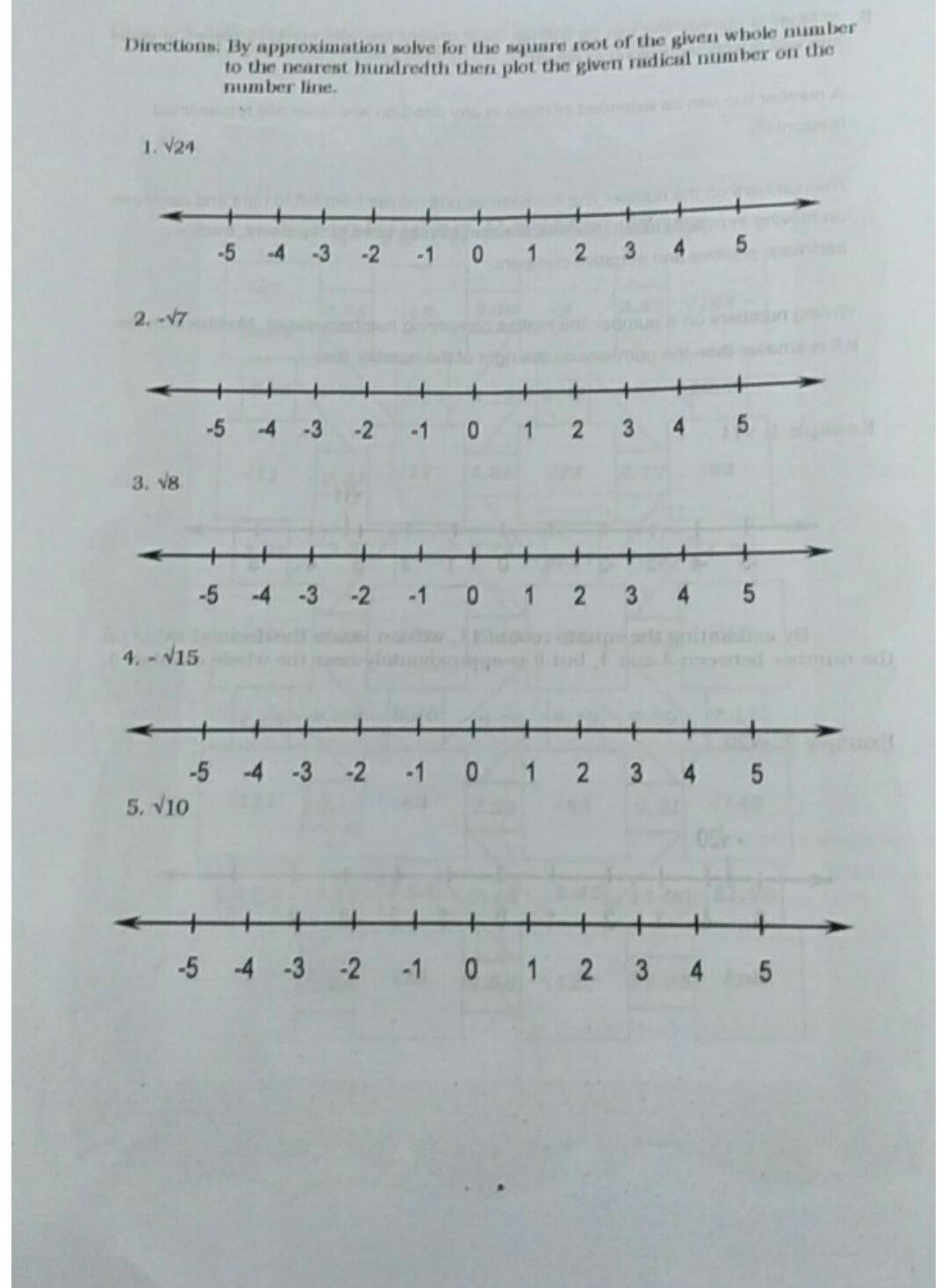Symbol
Problem$Directions$ A. Tell whether the given radicals are rational or irrational. Write your answer on the space provided. $1$ $\sqrt{49}$ $2$ $\sqrt{26}$ $3.\sqrt{64}$ $4$ $\sqrt{120}$ $5.\sqrt{1024}$
7th-9th grade
Other
Search count: 106
SolutionQanda teacher - honey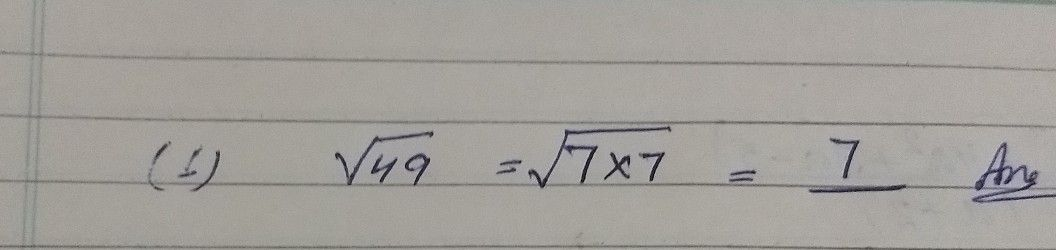Student
hi ma'am
my question is this rational or irrational ☺️Qanda teacher - honey
ok
I will sendStudent
thank you ma'am
i will wait
ma'am?Qanda teacher - honey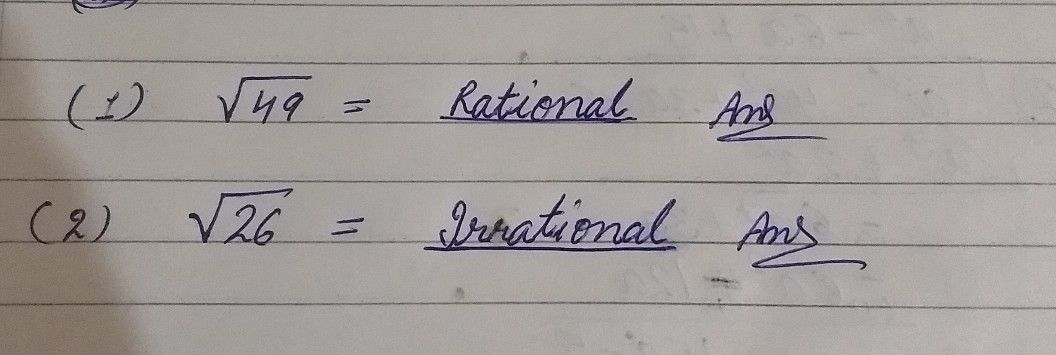Student
i need 3 left ma'amQanda teacher - honey
ok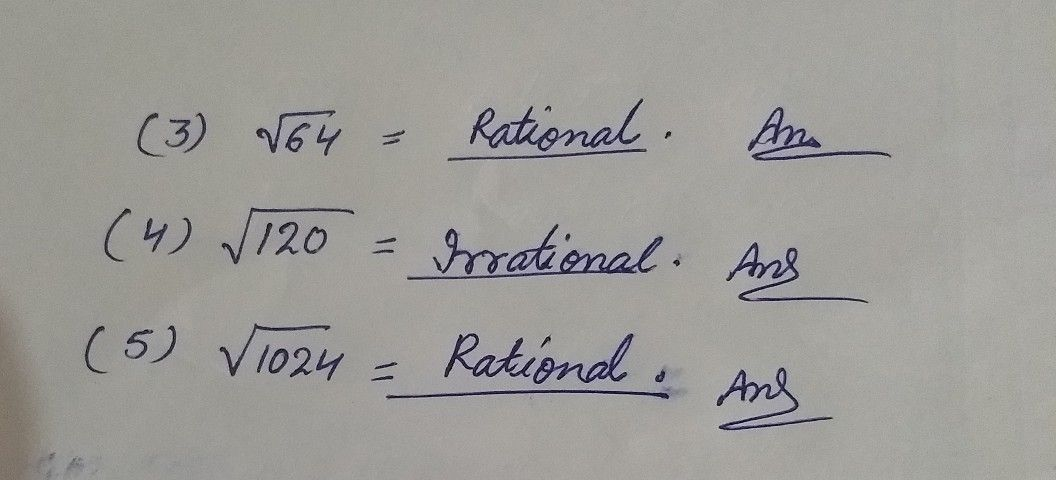Student
thank you ma'am this is my question 2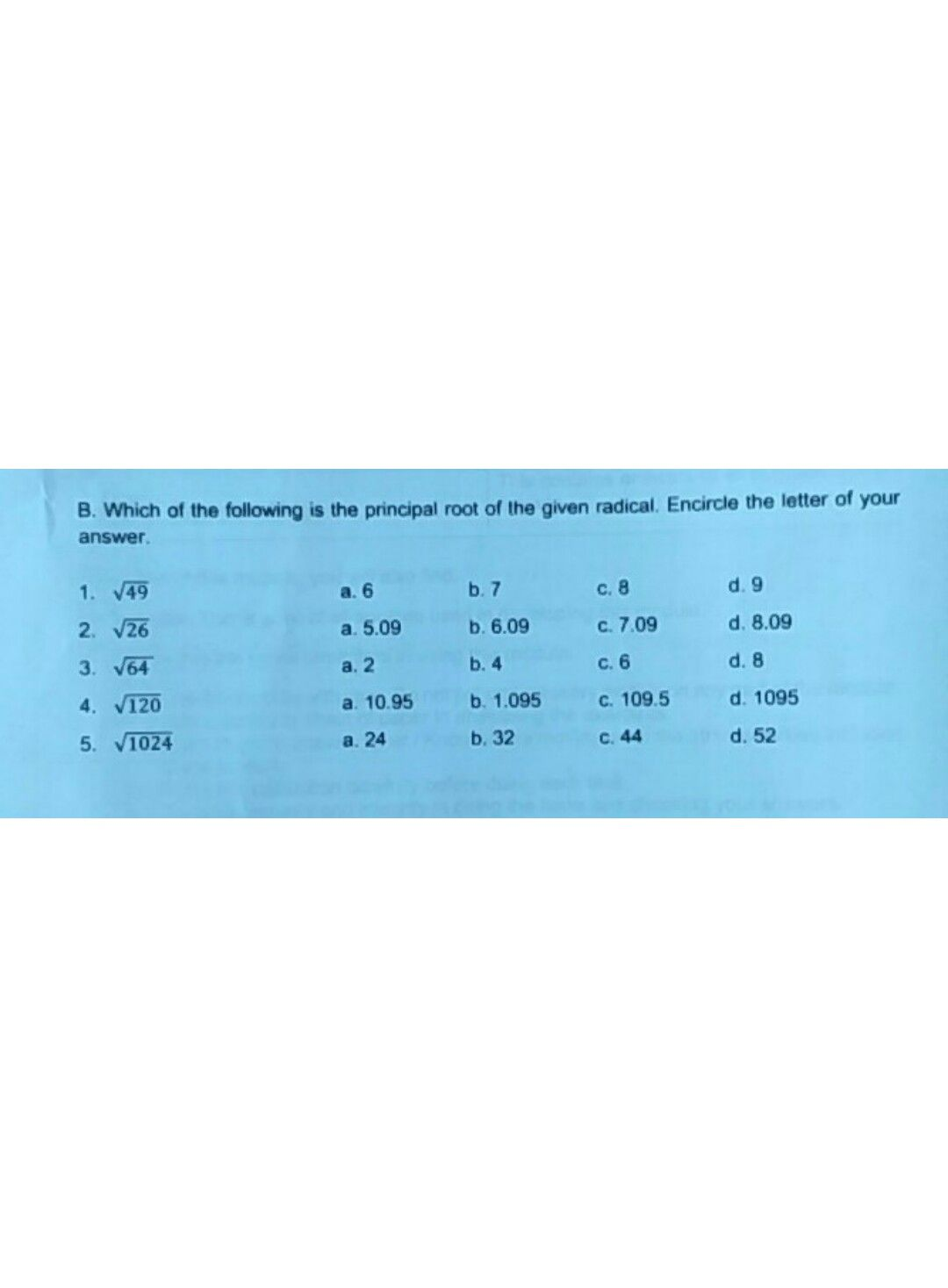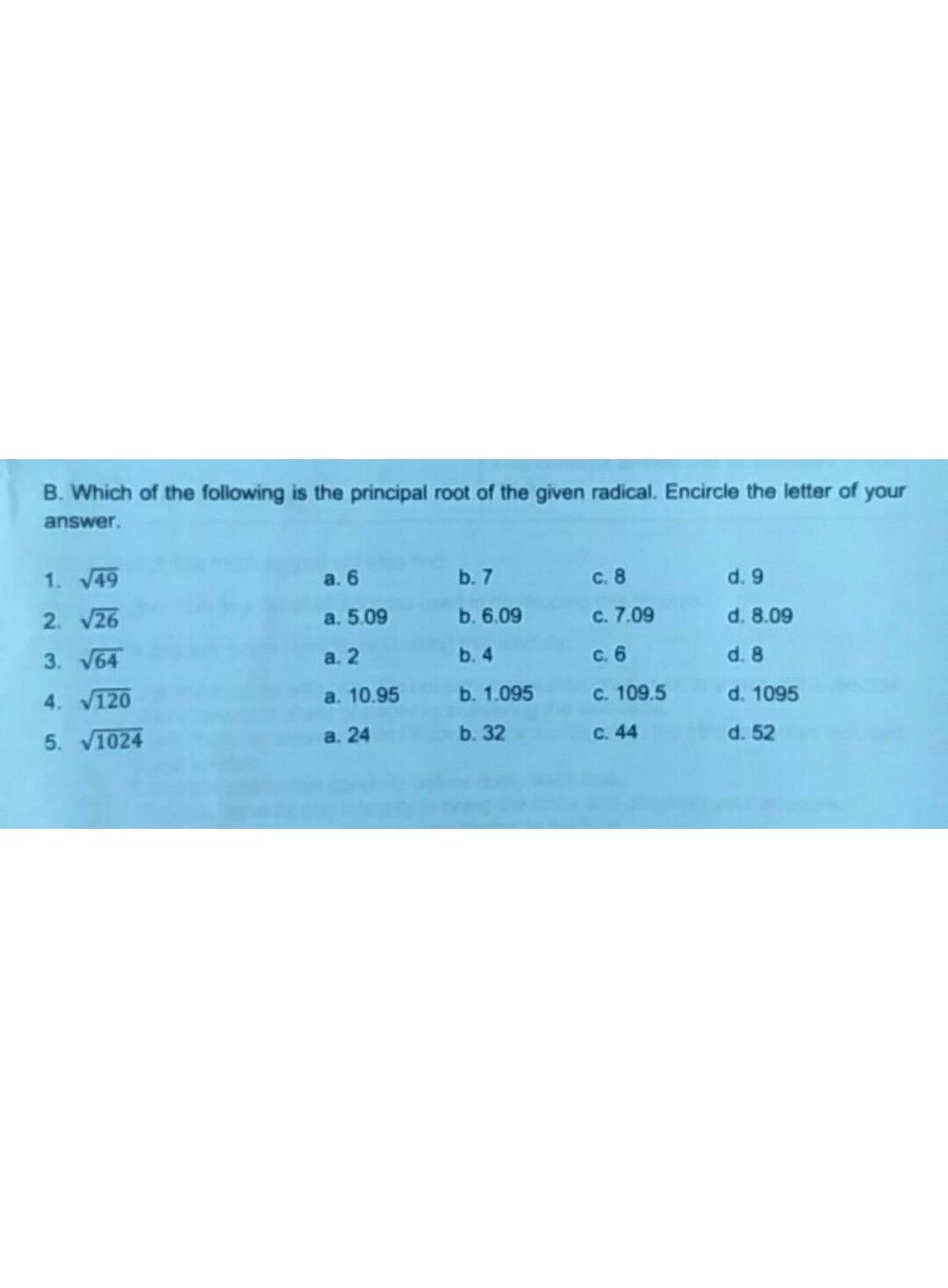ma'amQanda teacher - honey
ok waitStudent
ok ma'am i waitQanda teacher - honey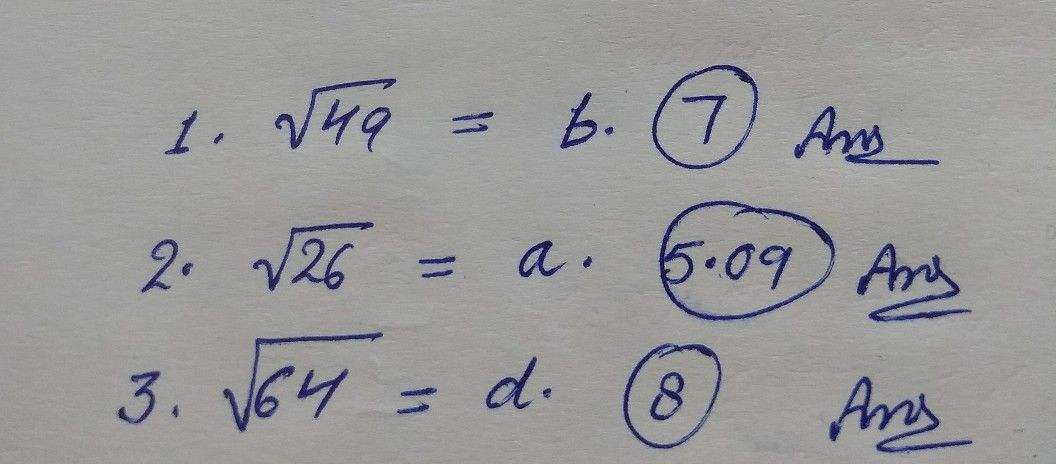Student
thanks for answer my question have questions to you again waitQanda teacher - honey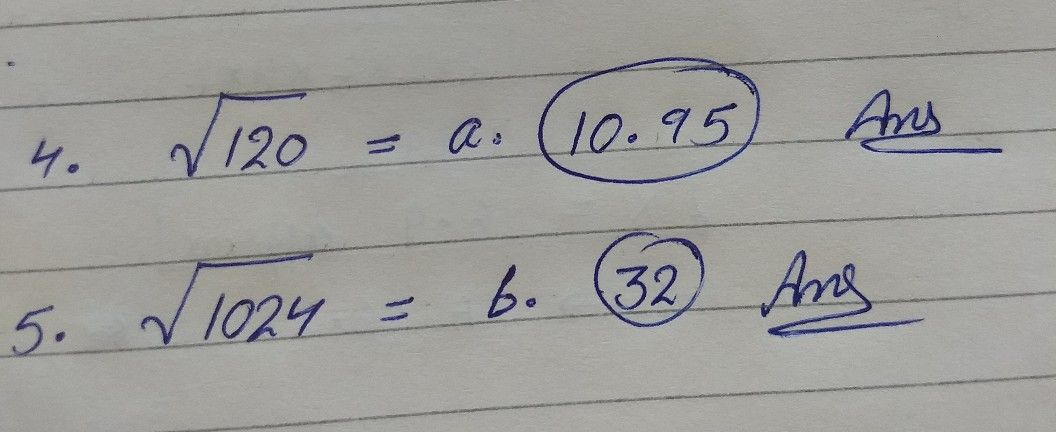if you understand give me five star for evolution of solutionStudent